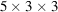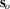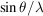International Tables for Crystallography (2006). Vol. F, ch. 2.1, pp. 45-63   | 1 | 2 | https://doi.org/10.1107/97809553602060000658

## Contents

• 2.1. Introduction to basic crystallography  (pp. 45-63)
• 2.1.1. Crystals  (pp. 45-46) | html | pdf |
• 2.1.2. Symmetry  (pp. 46-47) | html | pdf |
• 2.1.3. Point groups and crystal systems  (pp. 47-52) | html | pdf |
• 2.1.4. Basic diffraction physics  (pp. 52-57) | html | pdf |
• 2.1.4.1. Diffraction by one electron  (pp. 52-53) | html | pdf |
• 2.1.4.2. Scattering by a system of two electrons  (p. 53) | html | pdf |
• 2.1.4.3. Scattering by atoms  (pp. 53-54) | html | pdf |
• 2.1.4.3.1. Scattering by one atom  (pp. 53-54) | html | pdf |
• 2.1.4.3.2. Scattering by a plane of atoms  (p. 54) | html | pdf |
• 2.1.4.4. Anomalous dispersion  (pp. 54-55) | html | pdf |
• 2.1.4.5. Scattering by a crystal  (pp. 55-56) | html | pdf |
• 2.1.4.6. The structure factor  (pp. 56-57) | html | pdf |
• 2.1.5. Reciprocal space and the Ewald sphere  (pp. 57-58) | html | pdf |
• 2.1.6. Mosaicity and integrated reflection intensity  (pp. 58-59) | html | pdf |
• 2.1.7. Calculation of electron density  (pp. 59-60) | html | pdf |
• 2.1.8. Symmetry in the diffraction pattern  (pp. 60-61) | html | pdf |
• 2.1.9. The Patterson function  (pp. 61-62) | html | pdf |
• References | html | pdf |
• Figures
• Fig. 2.1.1.1. One unit cell with axes a , b and c   (p. 45) | html | pdf |
• Fig. 2.1.1.2. A set ofunit cells  (p. 45) | html | pdf |
• Fig. 2.1.1.3. A two-dimensional lattice withunit cells  (p. 46) | html | pdf |
• Fig. 2.1.1.4. Non-centred and centred unit cells  (p. 46) | html | pdf |
• Fig. 2.1.3.1. How to construct a stereographic projection  (p. 47) | html | pdf |
• Fig. 2.1.3.2. A rhombohedral unit cell  (p. 47) | html | pdf |
• Fig. 2.1.3.3. The 14 Bravais lattices  (p. 52) | html | pdf |
• Fig. 2.1.4.1. The electric vector of a monochromatic and polarized X-ray beam is in the plane  (p. 53) | html | pdf |
• Fig. 2.1.4.2. The black dots are electrons  (p. 53) | html | pdf |
• Fig. 2.1.4.3. The direction of the incident wave is indicated byand that of the scattered wave by s   (p. 53) | html | pdf |
• Fig. 2.1.4.4. An Argand diagram for the scattering by two electrons  (p. 54) | html | pdf |
• Fig. 2.1.4.5. The atomic scattering factor f for carbon as a function of, expressed in units of the scattering by one electron  (p. 54) | html | pdf |
• Fig. 2.1.4.6. S is the X-ray source and D is the detector  (p. 54) | html | pdf |
• Fig. 2.1.4.7. Schematic picture of the Argand diagram for the scattering by atoms in a plane  (p. 55) | html | pdf |
• Fig. 2.1.4.8. The atomic scattering factor as a vector in the Argand diagram  (p. 55) | html | pdf |
• Fig. 2.1.4.9. X-ray diffraction by a crystal is, in Bragg's conception, reflection by lattice planes  (p. 56) | html | pdf |
• Fig. 2.1.4.10. The Wilson plot for phospholipase A 2 with data to 1.7 Å resolution  (p. 57) | html | pdf |
• Fig. 2.1.5.1. A two-dimensional real unit cell is drawn together with its reciprocal unit cell  (p. 58) | html | pdf |
• Fig. 2.1.5.2. The circle is, in fact, a sphere with radius(p. 58) | html | pdf |
• Fig. 2.1.7.1. An Argand diagram for the structure factors of the two members of a Friedel pair  (p. 60) | html | pdf |
• Fig. 2.1.9.1. ( a ) A two-dimensional unit cell with two atoms  (p. 62) | html | pdf |
• Tables
• Table 2.1.2.1. The most common space groups for protein crystals  (p. 46) | html | pdf |
• Table 2.1.3.1. The 11 enantiomorphic point groups  (pp. 48-49) | html | pdf |
• Table 2.1.3.2. The 11 point groups with a centre of symmetry  (pp. 50-51) | html | pdf |
• Table 2.1.3.3. The icosahedral point group 532  (p. 51) | html | pdf |
• Table 2.1.3.4. The seven crystal systems  (p. 52) | html | pdf |
• Table 2.1.4.1. The position of the K α edge of different elements  (p. 54) | html | pdf |